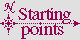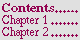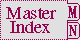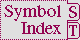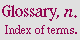#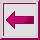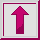## 6.1.3 Value Accumulation Clauses

The constructs collect, collecting, append, appending, nconc, nconcing, count, counting, maximize, maximizing, minimize, minimizing, sum, and summing, allow values to be accumulated in a loop.

The constructs collect, collecting, append, appending, nconc, and nconcing, designate clauses that accumulate values in lists and return them. The constructs count, counting, maximize, maximizing, minimize, minimizing, sum, and summing designate clauses that accumulate and return numerical values.

During each iteration, the constructs collect and collecting collect the value of the supplied form into a list. When iteration terminates, the list is returned. The argument var is set to the list of collected values; if var is supplied, the loop does not return the final list automatically. If var is not supplied, it is equivalent to supplying an internal name for var and returning its value in a finally clause. The var argument is bound as if by the construct with. No mechanism is provided for declaring the type of var; it must be of type list.

The constructs append, appending, nconc, and nconcing are similar to collect except that the values of the supplied form must be lists.

* The append keyword causes its list values to be concatenated into a single list, as if they were arguments to the function append.

* The nconc keyword causes its list values to be concatenated into a single list, as if they were arguments to the function nconc.

The argument var is set to the list of concatenated values; if var is supplied, loop does not return the final list automatically. The var argument is bound as if by the construct with. A type cannot be supplied for var; it must be of type list. The construct nconc destructively modifies its argument lists.

The count construct counts the number of times that the supplied form returns true. The argument var accumulates the number of occurrences; if var is supplied, loop does not return the final count automatically. The var argument is bound as if by the construct with to a zero of the appropriate type. Subsequent values (including any necessary coercions) are computed as if by the function 1+. If into var is used, a type can be supplied for var with the type-spec argument; the consequences are unspecified if a nonnumeric type is supplied. If there is no into variable, the optional type-spec argument applies to the internal variable that is keeping the count. The default type is implementation-dependent; but it must be a supertype of type fixnum.

The maximize and minimize constructs compare the value of the supplied form obtained during the first iteration with values obtained in successive iterations. The maximum (for maximize) or minimum (for minimize) value encountered is determined (as if by the function max for maximize and as if by the function min for minimize) and returned. If the maximize or minimize clause is never executed, the accumulated value is unspecified. The argument var accumulates the maximum or minimum value; if var is supplied, loop does not return the maximum or minimum automatically. The var argument is bound as if by the construct with. If into var is used, a type can be supplied for var with the type-spec argument; the consequences are unspecified if a nonnumeric type is supplied. If there is no into variable, the optional type-spec argument applies to the internal variable that is keeping the maximum or minimum value. The default type is implementation-dependent; but it must be a supertype of type real.

The sum construct forms a cumulative sum of the successive primary values of the supplied form at each iteration. The argument var is used to accumulate the sum; if var is supplied, loop does not return the final sum automatically. The var argument is bound as if by the construct with to a zero of the appropriate type. Subsequent values (including any necessary coercions) are computed as if by the function +. If into var is used, a type can be supplied for var with the type-spec argument; the consequences are unspecified if a nonnumeric type is supplied. If there is no into variable, the optional type-spec argument applies to the internal variable that is keeping the sum. The default type is implementation-dependent; but it must be a supertype of type number.

If into is used, the construct does not provide a default return value; however, the variable is available for use in any finally clause.

Certain kinds of accumulation clauses can be combined in a loop if their destination is the same (the result of loop or an into var) because they are considered to accumulate conceptually compatible quantities. In particular, any elements of following sets of accumulation clauses can be mixed with other elements of the same set for the same destination in a loop form:

* collect, append, nconc

* sum, count

* maximize, minimize

```;; Collect every name and the kids in one list by using
;; COLLECT and APPEND.
(loop for name in '(fred sue alice joe june)
for kids in '((bob ken) () () (kris sunshine) ())
collect name
append kids)
=>  (FRED BOB KEN SUE ALICE JOE KRIS SUNSHINE JUNE)
```

Any two clauses that do not accumulate the same type of object can coexist in a loop only if each clause accumulates its values into a different variable.

## 6.1.3.5 Examples of SUM clause